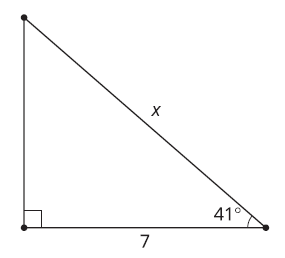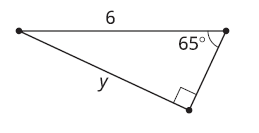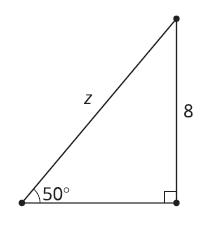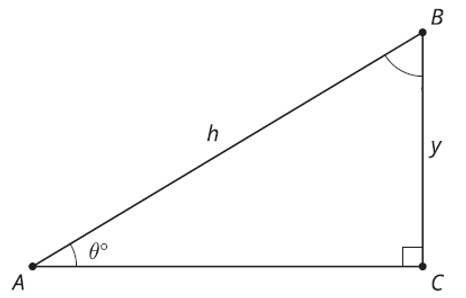# G.4.8.2 Twin Triangles

Topic:
Triangles

Show 2 different equations you could use to solve for x, then solve each for x.Show 2 different equations you could use to solve for y, then solve each for y.Show 2 different equations you could use to solve for z, then solve each for z.Write an expresson for the value of angle B using Ɵ. Write an equation using the value of angle A (Ɵ) that you could use to solve for y. Write an equation using the value of angle B (involving Ɵ) that you could use to solve for y.